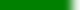The following is simply a conveniently formatted list of learning goals. More likely than not, some might be hard to assess through a quiz or exam. Students should prioritize their studying based on:

• how much time in lecture was spent discussing or understanding the topic,
• how much the topic was stressed in learning group assignments
• and the student's comfort level with the topic

# Assessable Learning Goals covered for Final Exam Learning GoalsKnow Fisher-Yates shuffling algorithm, be able to apply it to sampling tasks in simulations.Know Fisher-Yates shuffling algorithm, be able to present a convincing argument of its correctness.Know the reservoir sampling algorithm for selecting n random elements from an unkown sized population.Recall casino thieves, be able to write an algorithm that deals deterministic n-player 5-card poker handsKnow how to use accept/reject techniques for uniformly random (geometric) point generation.Know how to write Monte Carlo simulations for estimating a discrete data histogram of many events.Know how to write Monte Carlo simulations for estimating the Pr(A) of an event A.Know the pitfalls associated with common (naive) methods of random point generation.What unique characteristic of a system or simulation makes it Monte Carlo?Be able to implement a non-trivial DES using next event software architecture.Describe S, the system state. What does "system" refer to?How is the simulation clock different than the wall clock or an accelerated, virtual clock?Know the basic algorithm for executing a DES using next event methodology.Understand the basic steps in augmenting a pre-existing NES with additional system and meta events.What constraint(s) are placed on events being inserted into the event list?What is the difference between system events and meta events?How do you apply pRNG streams to achieve variance reduction in simulation experiements?Explain the values a, m, g() and x0 in a (Lehmer) PMMLCG; how is the next Uniform(0,1) random value calculated?Given a and m (a a full period multiplier), find the smallest and largest floating point values between 0 and 1 that can be generated.How is a (sub)stream of a Lehmer pRNG created?How is an xi+1 value converted from an integer to a real number in the interval (0,1)?What does the pRNG API routine Random() provide to a simulation writer?What is a full period multiplier and why is it important to Lehmer pRNGs?Be able to distinguish between finite horizon and infinite horizon system statistics.Be able to distinguish between transient and steady state system statistics.How do you create a confidence interval for a sample mean given the sample variance?How does autocorrelation affect the validity of calculating confidence intervals from batch means?How is the central limit theorem important in the interpretation of simulation results?How is the mean of a particular steady state statistic affected by the choice of b and k?Know the Batch Means Algorithm (8.4.1) and the definitions of variables b and k.What does a confidence interval mean? How would you explain it to your middle-school cousin?What is a distribution's `idf()`?What is special about outliers in the context of computer simulation results?Understand the interprettation and connection between a statistical test with "α=0.05" and a 95% confidence interval.How does serial correlation affect estimated mean and standard deviation?What is the difference between serial and paired correlation?Know what constitutes a histogram and how it is connected to CDFs.Given Welford's discrete and integral mean and variance equations (Thms 4.1.2, 4.1.4), be able to apply them to a set of data.Know that Welford's Equations exist, why they are superior to the "one-pass" algorithm common in statistical texts.Know which of the two (non Welford) standard equations for calculating s2 or s is flawed.Know how the naive adaption of the stationary arrival time algorithm fails when substituting λ with λ(t).Know that exponential interarrival times yields arrival times that are uniformly distributed.Know that uniform arrival times and uniform interarrivals are not the same thing.Know that valid computer simulations do not produce outliers. End of discussion.Know the inversion technique for non-stationary arrival times.Know the thinning technique for non-stationary arrival times (what is its downside?)Understand the mechanics of algorithm 8.1.2 which minimizes the number of simulation runs for alpha confidence intervals.What are consistency checks? How can they be used in V&V?What are the authors' five phases of simulation development?What is simulation validation?What is simulation verification?What is the computational model of a simulation?What is the conceptual model of a simulation?What is the specification model of a simulation?Know how to calculate traffic intensity and its connection to service rate.Understand how a FIFO SSQ simulation can be written in a simple while loop and how a_i and s_i can be manipulated for simple experiments.Understand the canonical SSQ and appreciate its broad application to computer simulation.Know the relationships between PMF/PDF and CDF for both discrete and continuous distributions.Know the relationships between PMF/PDF, CDF, and InvDF. How do you derive one from another? Can this always be done?Know the CDF search technique for creating discrete random variates.Know the Equilikely(a,b) random variate: the meaning of its parameters, pmf, and CDF.Know the Exponential(mu) random variate and the interpretation of its parameter mu in the context of arrival times.Know the F(x) inversion technique for constructing random variates. What is the requirement on F(x)?Know the Uniform(a,b) random variate: the meaning of its parameters, pdf, and CDF.Know the three techniques for constructing a discrete random variate.Know what the construction technique means for random variates (of which summation is one example).Understand the problems with the often used and always flawed `RandomInteger() mod SIZE` programming pattern.What does the parameter u in random variates represent? Computationally, how do we get a value for u in code?